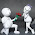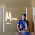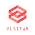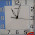## Index

 STA & SI Chapter1 Chapter2 Chapter3 Chapter4 Chapter5 Chapter6 Chapter7 Chapter8 Introduction Static Timing Analysis Clock Advance STA Signal Integrity EDA Tools Timing Models Other Topics

 Extraction & DFM Chapter1 Chapter2 Chapter3 Chapter4 Chapter5 Chapter6 Introduction Parasitic Interconnect Corner (RC Corner) Manufacturing Effects and Their Modeling Dielectric Layer Process Variation Other Topic

# How To Solve Setup and Hold Violation

 STA & SI:: Chapter 2: Static Timing Analysis 2.1 2.2 2.3a 2.3b 2.3c 2.4a Timing Paths Time Borrowing Basic Concept Of Setup-Hold Basic Concept of Setup-Hold Violation Examples:S-H Time/Violation Timing Path Delay 2.4b 2.4c 2.5a 2.5b 2.6a 2.6b Interconnect Delay Models Delay - Wire Load Model Maximum Clock Frequency Calculate “Max Clock Freq”-Examples Fix Setup-Hold Violation-1 Fix Setup-Hold Violation-2 2.6c 2.7a 2.7b 2.7c 2.8 Fix Setup-Hold Violation-3 Incr/Decr Delay Method-1 Incr/Decr Delay Method-2 Incr/Decr Delay Method-3 10 ways to fix Setup-Hold Violation.

Static Timing analysis is divided into several parts:

We have discussed few basics about the “Setup and Hold violation” in last few posts. Once designer’s figured out the number of setup and hold violation then their next challenge is: “How to fix these violations”.

EDA tools usually take care but still you have to provide the input (or say proper switch) to fix these violations. That means I can say that “Timing/Routing Tools are enough intelligent to solve most of the timing violation, but still Tools never be more intelligent than the human brain”. J
There are different ways to fix these issues and every way has the reason for that. So designers should know what exactly the reason of Issue and what are the different methods (priority wise) or at least different EDA’s switch for fixing those violation.

In this series we will discuss the following things one by one.
• Basic of Fixing the SETUP and HOLD violations.
• More Examples here. Very less theory.
• Few shortcuts/formula/tricks to find out whether these violations are fixable or not. And If fixable, then a rough idea where and how.
• Different ways to fix.
• Their basics or say physics/Engineering of using that method for fixing.
• Which method is good and in what scenario you can use them.
Before that- If still you have any doubt regarding the Setup and Hold then please refer following post.
What exactly is the setup and hold – please refer the previous blog.
What are setup and hold violation – please refer the previous blog.

### Basics of Fixing the “SETUP and HOLD”  violations.

Let’s start with following Diagram and consider this as common for next few examples.

In the following examples we will pick different values of Setup/Hold values of Capture FF and Combinational Path delay. Through these example we will study - How the setup and hold violations are dependent to each other and on the delay of the circuit. If these things are clear then it’s very easy for you to understand -- how can we fix the violations and if we are using any particular methods, then why?

#### Example 1:

 Specification of the FF Circuit Setup Hold Clock period Tck2q delay Net Delay Combinational Logic Delay 2ns 1ns 10ns 0ns (Ideal) 0ns (Ideal) 0.5ns

Let’s discuss the flow of the data from FF1 to FF2
• Data is going to launch from FF1 at +ive Clock Edge at 0ns and it will reach to FF2 after 0.5ns (combinational logic delay only).
• This data is going to capture at FF2 at +ive Clock Edge at 10ns.
• As per the Setup definition, data should be stable 2ns (Setup time of FF2) at FF2 before the +ive Clock Edge (which is at 10ns)
• In the above case – data become stable 9.5ns before the Clock edge at 10ns (10ns – 0.5ns). That means it satisfy the Setup condition. NO SETUP VIOLATION.
• At the FF1 – second set of data is going to launch at t=10ns and it will reach the FF2 in another 0.5ns, means at t=10.5ns.
• This second set of data is going to update/override the first set of data.
• As per the Hold Definition, data should be stable till 1ns (Hold time of FF2) at FF2 after the clock edge (which is at t=10ns)
• In the above case – first set of data is going to override by second set of data at 10.5ns (means just after 0.5ns of the +ive Clock edge at FF2). This means it is not satisfying the hold condition. HOLD VIOLATION.
To fix this Hold violation – we have to increase the delay of the Data path so that the second set of data should not reach before t=11ns (10ns+1ns). That means the minimum delay of the Combinational Logic Path should be 1ns for NO HOLD VIOLATION.
That means if you want to fix the HOLD violation, you can increase the Delay of the Data path by any method (we will discuss all those methods in detail – Just keep small patience :) ).

But it doesn’t mean that you can increase the Delay by any Value. Let’s assume that you have increased the delay of combinational path by adding extra buffer (with delay of 8.5ns).  Now new specifications are

 Specification of the FF Circuit Setup Hold Clock period Tck2q delay Net Delay Combinational Logic Delay 2ns 1ns 10ns 0ns (Ideal) 0ns (Ideal) =0.5ns+8.5ns=9ns

As per the Setup definition, data should be stable 2ns (Setup time of FF2) before the Clock Edge (at FF2 which is at 10ns) and with the updated specification – data will be stable at t=9ns, just 1ns before the Clock edge at t=10ns at FF2.  That means it is not satisfying the Setup condition. SETUP VIOLATION.

Since Data path delay is more than 1ns, there is NO HOLD VIOLATION (just we have discussed few paragraph above)

So it means that if we want to fix the setup violation, the Delay of the combinational path should not be more then 8ns (10ns – 2ns). Means 8ns is the maximum value of the Delay of the Combinational Logic path for NO SETUP VIOLATION.

So we can generalize this –
For NO HOLD and SETUP VIOLATION, the delay of the path should be in between 1ns and 8ns.
OR

For Violation free Circuit:

Min delay of Combinational path > Hold time of Capture FF.
Max delay of Combinational path < Clock Period - Setup time of Capture FF.

#### Example 2:

 Specification of the FF Circuit Setup Hold Clock period Tck2q delay Net Delay Combinational Logic Delay 6ns 5ns 10ns 0ns (Ideal) 0ns (Ideal) 0.5ns

Flow of the data from FF1 to FF2:

• Data is going to launch from FF1 at Clock Edge at 0ns and it will reach to FF2 after 0.5ns (combinational logic delay only).
• This data is going to capture at FF2 at Clock Edge at 10ns.
• As per the Setup definition, data should be stable 6ns (Setup time of FF2) before the Clock Edge (which is at 10ns)
• In the above case – data become stable 9.5ns before the Clock edge at 10ns (10ns – 0.5ns). That means it satisfy the Setup condition. NO SETUP VIOLATION.
• At the FF1 – second set of data is going to launch at t=10ns and it will reach the FF1 in another 0.5ns, means at t=10.5ns.
• This second set of data is going to update/override the first set of data.
• As per the Hold Definition, data should be stable till 5ns (Hold time of FF2) after the clock edge (which is at t=10ns) at FF2
• In the above case – first set of data is going to override by second set of data at 10.5ns (means just after 0.5ns of the Clock edge at FF2). This means it is not satisfying the hold condition. HOLD VIOLATION.
To fix this Hold violation – (As per the previous example) we may increase the delay of the Data path, so that the second set of data should not reach before t=15ns (10ns+5ns). That means the minimum delay of the Combinational Logic Path should be 5ns for NO HOLD VIOLATION.

But Now if you will verify the Setup condition once again (with combination delay of 5ns- which we have assumed for fixing the hold violation) then you come to know that data is going to stable only after 5ns (means 10ns-5ns = 5ns before the clock edge at t-10ns). But as per the setup condition data should be stable before 6ns. So it means now it’s not satisfying Setup Condition. Means SETUP VIOLATION.

So in this scenario, we can’t fix the setup and hold violation at the same time by adjusting the delay in the combinational logic.

You can also see it directly with the help of minimum and maximum value of combinational delay.

Min delay > Hold time of Capture FF (means 5ns)
Max Delay < Clock Period – Setup time of capture FF (Means 10ns – 6ns = 4ns)

So Min delay > 5ns and Max Delay < 4ns which is not possible.

Now the point is how to fix these violations? Actually this is a non-fixable issue until you just change the clock frequency or replace the FF with lesser setup/hold value. J

Let me explain this.
Min delay has dependence only on Hold time, which is fixed for a particular FF.
Max delay has dependence on 2 parameters – Clock Period and Setup time - where Setup time is fixed for a particular FF.
So if you can change the FF with lower setup/hold violation, then you can fix this issue. But in case if that’s not possible then we have to change the Clock period.

In case we are changing the Clock period:

Keep --  Min delay >= 5ns ( No HOLD Violation)

Setup violation is by 6ns-5ns =1ns (6ns= Setup time and 5ns = combinational delay). What if we will increase the Clock period by 1ns. Means New clock period should be > 11ns.

So for Clock Period 11ns:

Max delay <= Clock period (11ns) – Setup time (6ns) =5ns.

Now - Max Delay=Min Delay = 5ns. (Neither Hold nor Setup Violation.)

We can generalize-

For Violation Free Circuit

Clock Period >= Setup time + Hold time.

#### Summary of this Post:

Min delay of Combinational path > Hold time of Capture FF.
Max delay of Combinational path < Clock Period - Setup time of Capture FF.
Clock Period >= Setup time + Hold time.

In the next part we will discuss few more examples with more restrictions. Like-
• What if we can’t reduce the Delay in the Data path?

1.Good One Puneet!

2.Very Nice Work Punnet.

3.superb....

4.5.6.In general hold value will be greater than setup

7.Really good info.

8.Really Helpful Thanks. Keep it up!

9.Excellent way of explaining.

10.11.very good , thanks for technical support

12.among analog nets and clock nets. what is the preference to be given?

13.Hi,
These are the circuits with positive edge flip flops, Can u give some examples with negative edged triggered flip flop,2 phased pulsed latch ,where the set up and hold time is violated